## Organic chemistry - some basic principles and techniques Quiz-26

IIT JEE exam which consists of JEE Main and JEE Advanced is one of the most important entrance exams for engineering aspirants. The exam is held for candidates who are aspiring to pursue a career in the field of engineering and technical studies. Chemistry is important because everything you do is chemistry! Even your body is made of chemicals. Chemical reactions occur when you breathe, eat, or just sit there reading. All matter is made of chemicals, so the importance of chemistry is that it's the study of everything..

Q1.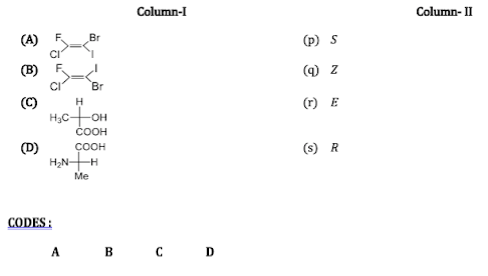•  r q s p
•  s p q r
•  q r s p
•  s p r q
Solution

(a→ q) (Z); two higher priority groups Cl and I are on the same side (b→ r) (E); Two higher priority groups Cl and I are on the opposite sides (c→ s) (R); priority order: -OH>-COOH>-CH_3>H H is on the vertical line; sequence is clockwise and hence (R) configuration (d→ p) (S); priority order : -NH_2>-COOH>Me>H H is on the horizontal line, with one interchange of H and Me; configuration is clockwise, so (S) configuration (odd number of interchanges)
Q2.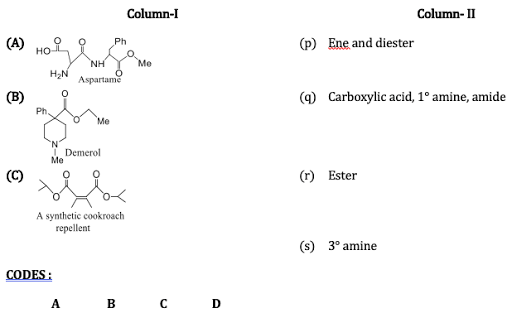•  P q r,s
•  p,q r s
•  r q,s p
•  q,r r,s p
Solution

All are self-explanatory

Q3. Which of the following compounds shows resonance?
•  Ethyne
•  Ethane
•  Touene
•  Cyclohexene
Solution
Toluene shows resonance due to delocalization of π-electrons

Q4. Which one of the halide is most reactive for S_N 2 reaction?
•  C_6 H_5 CH_2 Cl
•  CH_3-O-CH_2-Cl
•  CH_2=CH-CH_2-Cl
•  CH_3-CO-CH_2-Cl
Solution
Substrate having carbonyl group on β carbon is the excellent substrate for S_N 2 reaction

Q5. When 0.35 g of an organic compound is heated with HNO_3 and AgNO_3 in a Carius tube, it gives 0.70 g of silver chloride. The percentage of chloride in the compound is
•  54.8%
•  49.47%
•  34.6%
•  25.85%
Solution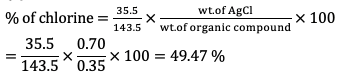Q6. What is the molecular formula of a compound, its empirical formula is CH_2 O and its molecular weight is 90°?
•  C_3 H_6 O_3
•  C_6 H_6 O_3
• C_4 H_8 O_4
•  C_2 H_6 O_2
Solution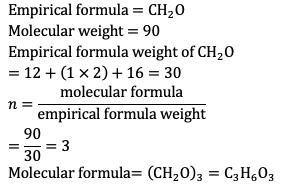Q7. The principal functional group in (A) us:
•  Phenyl
•  -COOH group
•  Isopropyl
•  Both (a) and (b)
Solution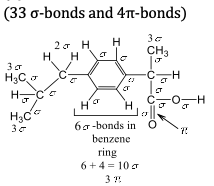Q8. The principal functional group in (A) is:
•  Phenyl
•  -COOH
•  Ester
•  All
Solution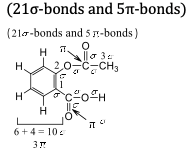Q9. Which of the following compounds will give positive Lassaigne's test for nitrogen
•  NH_2 OH
•  NH_2 NH_2
•  KCN
•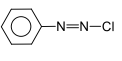Solution

Positive Lassaigne's test is given by the compound which contains both C and N. Moreover, this test is not given by diazonium compounds because they lose N_2 on heating much before they react with Na Hence the answer is (c)
Q10. Volume of O_2used is:
•  70 ml
•  75 ml
•  80 ml
• 85 ml
Solution
Volume of O_2used =(400×20)/100=80 ml#### Written by: AUTHORNAME

AUTHORDESCRIPTION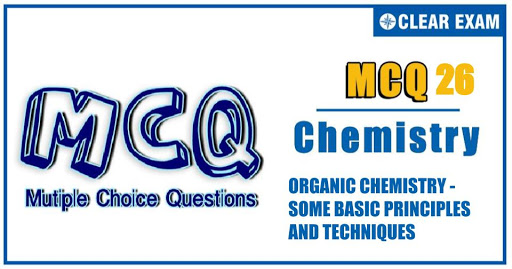## Want to know more

Please fill in the details below:

## Latest NEET Articles\$type=three\$c=3\$author=hide\$comment=hide\$rm=hide\$date=hide\$snippet=hide

Name

ltr
item
BEST NEET COACHING CENTER | BEST IIT JEE COACHING INSTITUTE | BEST NEET & IIT JEE COACHING: ORGANIC CHEMISTRY - SOME BASIC PRINCIPLES AND TECHNIQUES Quiz-26
ORGANIC CHEMISTRY - SOME BASIC PRINCIPLES AND TECHNIQUES Quiz-26